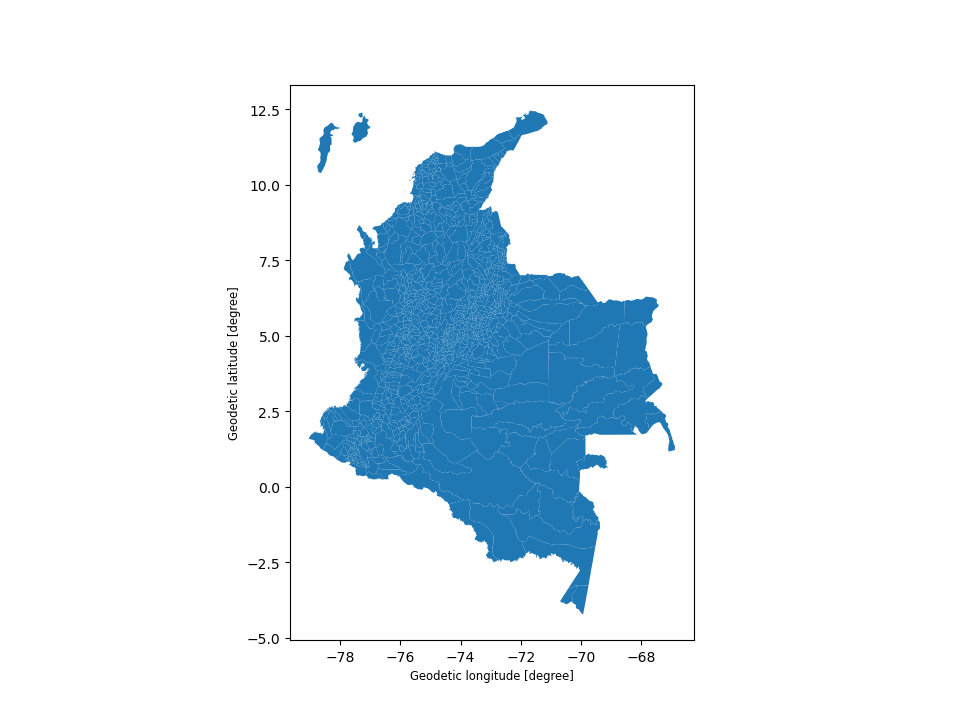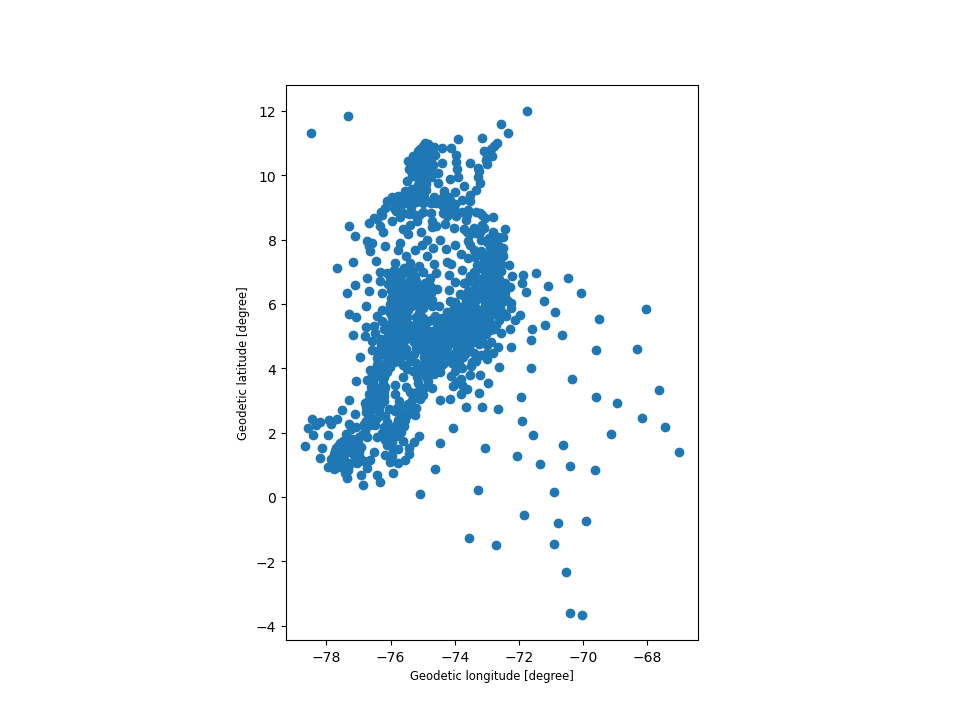# Data structures#

GeoPandas implements two main data structures, a `GeoSeries` and a `GeoDataFrame`. These are subclasses of `pandas.Series` and `pandas.DataFrame`, respectively.

## GeoSeries#

A `GeoSeries` is essentially a vector where each entry in the vector is a set of shapes corresponding to one observation. An entry may consist of only one shape (like a single polygon) or multiple shapes that are meant to be thought of as one observation (like the many polygons that make up the State of Hawaii or a country like Indonesia).

GeoPandas has three basic classes of geometric objects (which are actually shapely objects):

• Points / Multi-Points

• Lines / Multi-Lines

• Polygons / Multi-Polygons

Note that all entries in a `GeoSeries` do not need to be of the same geometric type, although certain export operations will fail if this is not the case.

### Overview of attributes and methods#

The `GeoSeries` class implements nearly all of the attributes and methods of Shapely objects. When applied to a `GeoSeries`, they will apply elementwise to all geometries in the series. Binary operations can be applied between two `GeoSeries`, in which case the operation is carried out elementwise. The two series will be aligned by matching indices. Binary operations can also be applied to a single geometry, in which case the operation is carried out for each element of the series with that geometry. In either case, a `Series` or a `GeoSeries` will be returned, as appropriate.

A short summary of a few attributes and methods for GeoSeries is presented here, and a full list can be found in the GeoSeries API reference. There is also a family of methods for creating new shapes by expanding existing shapes or applying set-theoretic operations like “union” described in Geometric manipulations.

## GeoDataFrame#

A `GeoDataFrame` is a tabular data structure that contains a `GeoSeries`.

The most important property of a `GeoDataFrame` is that it always has one `GeoSeries` column that holds a special status. This `GeoSeries` is referred to as the `GeoDataFrame`’s “geometry”. When a spatial method is applied to a `GeoDataFrame` (or a spatial attribute like `area` is called), this commands will always act on the “geometry” column.

The “geometry” column – no matter its name – can be accessed through the `geometry` attribute (`gdf.geometry`), and the name of the `geometry` column can be found by typing `gdf.geometry.name`.

A `GeoDataFrame` may also contain other columns with geometrical (shapely) objects, but only one column can be the active geometry at a time. To change which column is the active geometry column, use the `GeoDataFrame.set_geometry()` method.

An example using the `geoda.malaria` dataset from `geodatasets` containing the counties of Colombia:

```In : import geodatasets

Out:
0   1  COLOMBIA  ...  61773  POLYGON ((-71.32639 11.84789, -71.33579 11.855...
1   2  COLOMBIA  ...  36465  POLYGON ((-72.42191 11.79824, -72.41980 11.795...
2   3  COLOMBIA  ...  18368  POLYGON ((-72.18910 11.52420, -72.18330 11.532...
3   4  COLOMBIA  ...   7566  POLYGON ((-72.63800 11.36790, -72.62590 11.349...
4   5  COLOMBIA  ...   9343  POLYGON ((-74.77489 10.93158, -74.77530 10.933...

[5 rows x 51 columns]

# Plot countries
In : colombia.plot(markersize=.5);
```Currently, the column named “geometry” with county borders is the active geometry column:

```In : colombia.geometry.name
Out: 'geometry'
```

You can also rename this column to “borders”:

```In : colombia = colombia.rename_geometry('borders')

In : colombia.geometry.name
Out: 'borders'
```

Now, you create centroids and make it the geometry:

```In : colombia['centroid_column'] = colombia.centroid

In : colombia = colombia.set_geometry('centroid_column')

In : colombia.plot();
```Note: A `GeoDataFrame` keeps track of the active column by name, so if you rename the active geometry column, you must also reset the geometry:

```gdf = gdf.rename(columns={'old_name': 'new_name'}).set_geometry('new_name')
```

Note 2: Somewhat confusingly, by default when you use the `read_file()` command, the column containing spatial objects from the file is named “geometry” by default, and will be set as the active geometry column. However, despite using the same term for the name of the column and the name of the special attribute that keeps track of the active column, they are distinct. You can easily shift the active geometry column to a different `GeoSeries` with the `set_geometry()` command. Further, `gdf.geometry` will always return the active geometry column, not the column named `geometry`. If you wish to call a column named “geometry”, and a different column is the active geometry column, use `gdf['geometry']`, not `gdf.geometry`.

### Attributes and methods#

Any of the attributes calls or methods described for a `GeoSeries` will work on a `GeoDataFrame` – effectively, they are just applied to the “geometry” `GeoSeries`.

However, `GeoDataFrames` also have a few extra methods for input and output which are described on the Reading and writing files page and for geocoding with are described in Geocoding.

## Display options#

GeoPandas has an `options` attribute with currently a single configuration option to control:

```In : import geopandas

In : geopandas.options
Out:
Options(
display_precision: None [default: None]
The precision (maximum number of decimals) of the coordinates in the
WKT representation in the Series/DataFrame display. By default (None),
it tries to infer and use 3 decimals for projected coordinates and 5
decimals for geographic coordinates.
use_pygeos: False [default: False]
Whether to use PyGEOS to speed up spatial operations. The default is
True if PyGEOS is installed, and follows the USE_PYGEOS environment
variable if set.
io_engine: None [default: None]
The default engine for ``read_file`` and ``to_file``. Options are
'pyogrio' and 'fiona'.
)
```

The `geopandas.options.display_precision` option can control the number of decimals to show in the display of coordinates in the geometry column. In the `colombia` example of above, the default is to show 5 decimals for geographic coordinates:

```In : colombia['centroid_column'].head()
Out:
0    POINT (-71.74594 12.00885)
1    POINT (-72.56514 11.58174)
2    POINT (-72.35203 11.32204)
3    POINT (-73.14121 11.15251)
4    POINT (-74.64555 10.88454)
Name: centroid_column, dtype: geometry
```

If you want to change this, for example to see more decimals, you can do:

```In : geopandas.options.display_precision = 9